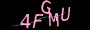×

# 收藏15个jquery小技巧，您值得拥有！！### 1、回到顶部按钮

```// Back to top
\$('.top').click(function (e) {
e.preventDefault();
\$('html, body').animate({scrollTop: 0}, 800);
});
<!-- Create an anchor tag -->
<a class="top" href="#">Back to top</a>```

### 2、图片预加载

```\$.preloadImages = function () {
for (var i = 0; i < arguments.length; i++) {
\$('<img>').attr('src', arguments[i]);
}
};

### 3、判断图片是否加载完

```\$('img').load(function () {
});```

### 4、自动修补破损图像

```\$('img').on('error', function () {
if(!\$(this).hasClass('broken-image')) {
}
});```

### 5、Hover切换class类

```\$('.btn').hover(function () {
}, function () {
\$(this).removeClass('hover');
});```

```\$('.btn').hover(function () {
\$(this).toggleClass('hover');
});```

### 6、禁用输入

`\$('input[type="submit"]').prop('disabled', true);`

`\$('input[type="submit"]').prop('disabled', false);`

### 7、停止正在加载的链接

```\$('a.no-link').click(function (e) {
e.preventDefault();
});```

```// Fade
\$('.btn').click(function () {
});
// Toggle
\$('.btn').click(function () {
\$('.element').slideToggle('slow');
});```

### 9、简单的手风琴

```// Close all panels
\$('#accordion').find('.content').hide();
// Accordion
var next = \$(this).next();
next.slideToggle('fast');
\$('.content').not(next).slideUp('fast');
return false;
});```

### 10、使两个DIV同等高度

`\$('.div').css('min-height', \$('.main-div').height());`

```var \$columns = \$('.column');
var height = 0;
\$columns.each(function () {
if (\$(this).height() > height) {
height = \$(this).height();
}
});
\$columns.height(height);```

```var \$rows = \$('.same-height-columns');
\$rows.each(function () {
\$(this).find('.column').height(\$(this).height());
});```

### 11、在浏览器标签/新窗口打开外部链接

```\$('a[href^="http"]').attr('target', '_blank');
\$('a[href^="//"]').attr('target', '_blank');
\$('a[href^="' + window.location.origin + '"]').attr('target', '_self');```

### 12、根据文本获取元素

```var search = \$('#search').val();
\$('div:not(:contains("' + search + '"))').hide();```

### 13、可见变化的触发

```\$(document).on('visibilitychange', function (e) {
if (e.target.visibilityState === "visible") {
console.log('Tab is now in view!');
} else if (e.target.visibilityState === "hidden") {
console.log('Tab is now hidden!');
}
});```

### 14、Ajax调用错误处理

```\$(document).ajaxError(function (e, xhr, settings, error) {
console.log(error);
});```

### 15、链式操作

jQuery允许通过链式操作来减轻反复查询DOM和创建多个jQuery对象的过程。比如下面是你的方法调用：

```\$('#elem').show();
\$('#elem').html('bla');
\$('#elem').otherStuff();```

```\$('#elem')
.show()
.html('bla')
.otherStuff();```

```var \$elem = \$('#elem');
\$elem.hide();
\$elem.html('bla');
\$elem.otherStuff();```

OK，希望大家看完以后，能应用到具体的项目当中。

https://www.jiangweishan.com/article/jqXiaoJqStuty.html

### 网友评论文明上网理性发言已有1人参与#### 评论列表

•发布于 2017-02-22 13:32:40
• 我已拥有，感谢分享。